• logistics回归
多人点赞 热门讨论
2021-04-11 11:22:12
import numpy as np

def sigmoid(x):
#请补全逻辑回归函数
return 1.0/(1+np.exp(-x))

def predict(x_test, weight):
if sigmoid(weight.T @ x_test) > 0.5:
return 1
return 0

#实现逻辑回归的训练
def fit(x, y):
m, n = x.shape
w = np.zeros(n).reshape((n,1))
y = y.reshape((m,1))
r = 0.001 #learning rate
cnt = 0
max_cnt = 10000 #设置最大迭代次数
t = 0.00001 #设置收敛条件
#利用梯度下降方法求最优解
#............................
for i in range(max_cnt):
# x:11*3 w:3*1
de = np.dot(x.T, y - sigmoid(np.dot(x,w)))
w += r*de

if np.linalg.norm(r*de)<=t:
break

# w:3*1
return w

if __name__ == '__main__':
x_train = np.array([
[1, 2.697, 6.254],
[1, 1.872, 2.014],
[1, 2.312, 0.812],
[1, 1.983, 4.990],
[1, 0.932, 3.920],
[1, 1.321, 5.583],
[1, 2.215, 1.560],
[1, 1.659, 2.932],
[1, 0.865, 7.362],
[1, 1.685, 4.763],
[1, 1.786, 2.523]
])
y_train = np.array([1, 0, 0, 1, 0, 1, 0, 0, 1, 0, 1])

weight = fit(x_train, y_train)
print(weight)
lis = []
#调用预测函数，输出x_train的预测结果，并输出准确率
for i in range(11):
lis.append(predict(x_train[i],weight))
print(lis)
n = 0 # 正确计数
for i in range(11):
if lis[i] == y_train[i]:
n+=1
print("正确率%s%%" %(n/11*100))

[[-3.29407748]
[ 0.02245258]
[ 0.79967711]]
[1, 0, 0, 1, 0, 1, 0, 0, 1, 1, 0]
正确率81.81818181818183%

更多相关内容
• logistics回归代码.rar,logistics回归代码,逻辑回归.m,logisticsB.png,logisticsA.png,population data.xls
• logistics回归分析算法python实现含训练集和数据集，logistics回归分析是一种线性回归分析模型
• 逻辑斯蒂回归，用Python语言写的，比较简单，在小数据集上准确率为100%，资源中包含完整代码及测试数据
• 用于logistics回归的测试数据集，其中logistics回归的Python实现和MATLAB实现在另一篇博文里
• 文章目录1、logistics回归2、多元分类总结 1、logistics回归   在处理分类问题时，使用线性回归难以将数据分好。如图，一个远处的样本点会大幅度影响线性回归曲线的走向，导致分类不准确。  因此要寻找一种适合...

## 1、logistics回归

在处理分类问题时，使用线性回归难以将数据分好。如图，一个远处的样本点会大幅度影响线性回归曲线的走向，导致分类不准确。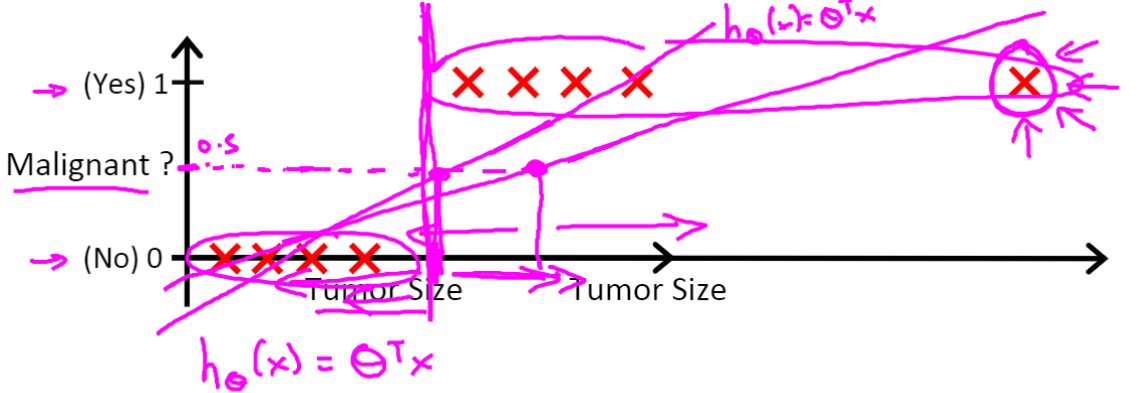因此要寻找一种适合分类的模型，比如logistics回归。这个方法用到了sigmoid函数，如图所示：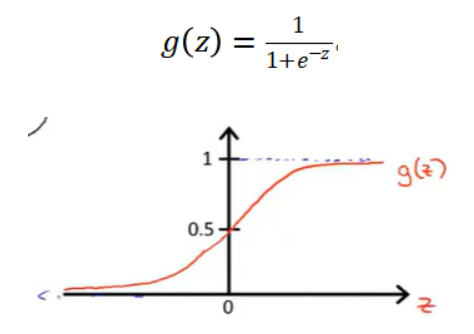当z趋于0+时，g(z)趋于1，当z趋于0-时，g(z)趋于0。这样就可以将数据区分为小于0和大于0两份。
但仅有这样一个分类函数是不够的，我们还需要一个完整的函数将两种数据分开，在分类流程中，这个函数被称为决策边界。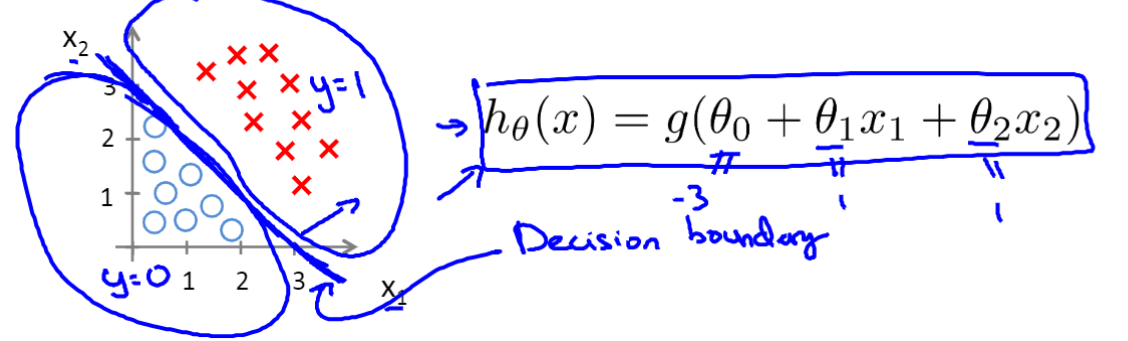如上图所示，将红叉和蓝圈分开来的直线，就是决策边界，这个带有参数的函数直线也是整个假设函数的一部分，将决策边界与sigmoid函数结合起来就是整个假设函数。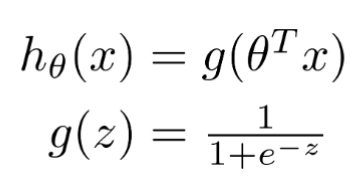有了新的假设函数，就有新的代价函数，新的代价函数如图所示：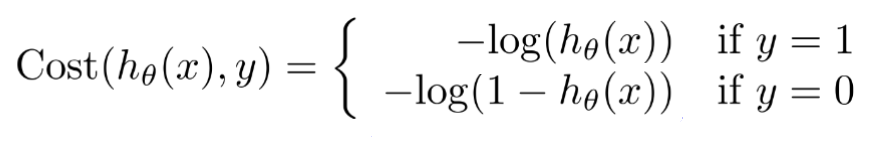因为h(x)取值在0和1之间，所以这个函数的意义就是鉴别h(x)是否等于y，如果不等，则有代价值，否则为0。通过数学手段简化后得到下面式子：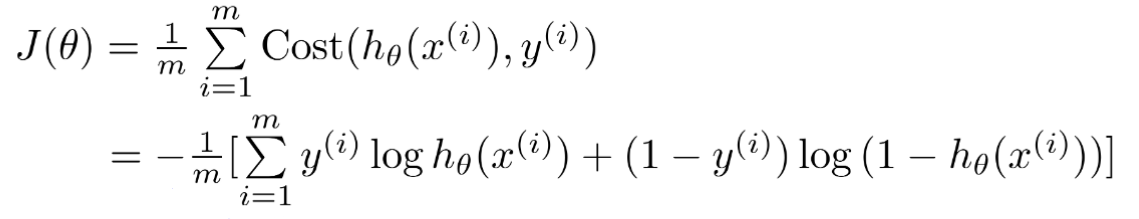得到代价函数之后，就可以通过梯度下降法优化参数求出最优解了。最终通过得到的模型预测输出。

## 2、多元分类

当分类涉及到两种以上的类型时，需要对分类算法进行改进。
具体的改造方法是构造多个分类器，分类器的数量等于需要划分的类型数量。每一个分类器都有一个决策边界，将其中一种类型与其他类型分开。这种做法可以使得在面对不同的输入时找到契合度最好的分类器。
当需要预测时，将输入分别放入所有分类器中，并选择得出的h(θ)值最大的分类器并得出结果。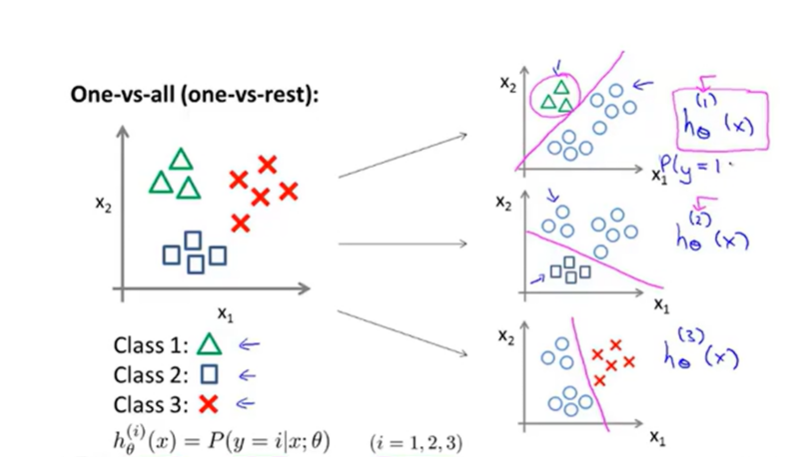# 总结

本章学习了logistics回归的原理。

展开全文• 数据回归-基于logistics回归的中小微企业信用评价研究.pdf
• 1.2 Logistic 回归的引入 Logistic 回归是概率非线性模型，用于处理二元分类结果，名为回归实为分类。下面给出一个二元分类的例子，如下图所示： 图中数据分成两类，打叉的一类用 y = 1 表示，另一类为圆圈用 y= 0...

目录

1.2 Logistic 回归的引入

1.3 分类预测函数问题的转化成求θ

1.4 梯度下降法求解θ

2.3 利用随机梯度上升法训练样本

### 1.2 Logistic 回归的引入

Logistic 回归是概率非线性模型，用于处理二元分类结果，名为回归实为分类。下面给出一个二元分类的例子，如下图所示：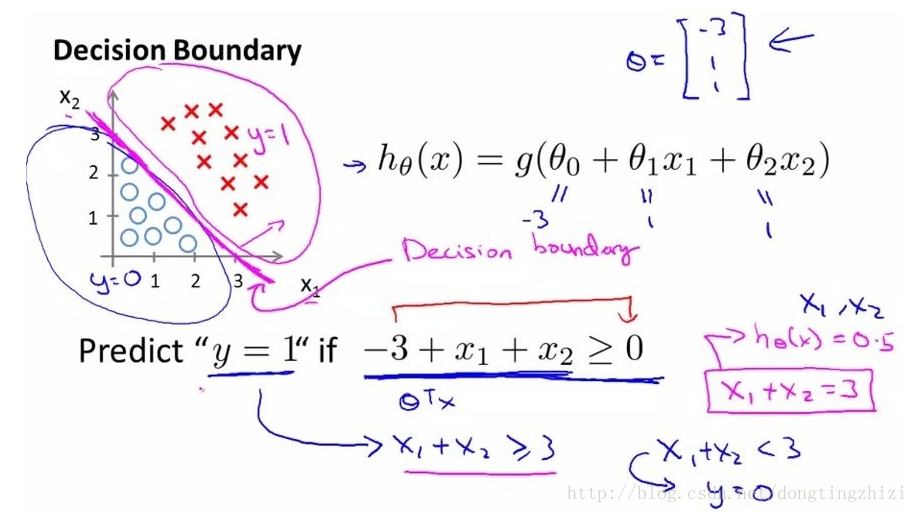图中数据分成两类，打叉的一类用 y = 1 表示，另一类为圆圈用 y= 0 表示。现在我们要对数据进行分类，要找到一个分类函数(边界线)，我们很容易得出一个线性函数对其分类：z = θ0 + θ1x1 + θ2x2 。但我们想要的函数应该是，能接受所有的输入然后预测类别。例如：在两个分类的情况下，函数输出 0 或 1。因此，我们就需要引入Sigmoid 函数，这个函数的性质可以满足要求。Sigmoid 函数：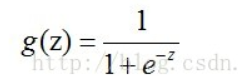Sigmoid 函数的值域为（0,1），且具有良好的从0 到 1 的跳跃性，如在两个不同的坐标尺度下的函数图形：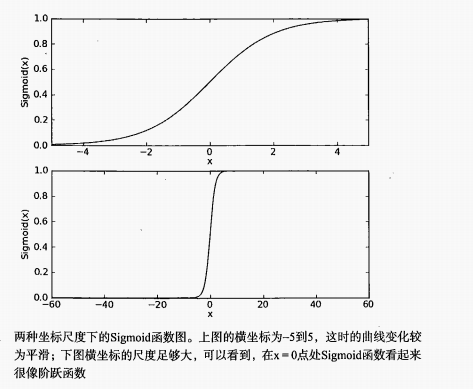所以，我们把线性方程和Sigmoid 函数结合起来就能解决问题。即 ：分类预测函数 hθ (x) = g( θ0 + θ1x1 + θ2x2) .我们就可以对样本数据进行分类，如下图所示：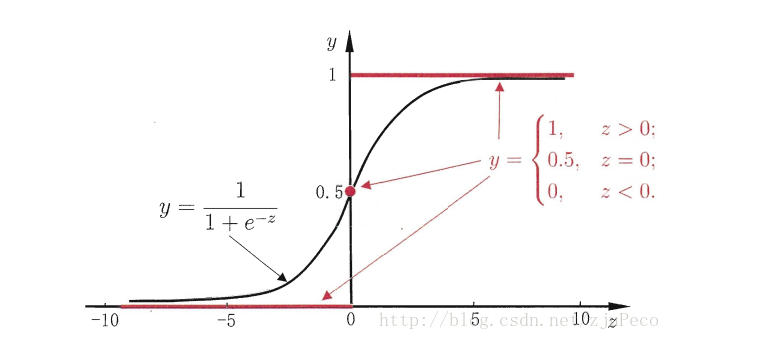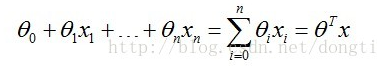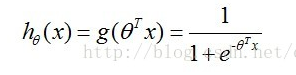其中，θT 是向量 θ (θ0, θ1,... ,θn) 的转置，向量 x是 ( x0 , x1 ,... , xn)，x0 =1，这是便于计算。

### 1.3 分类预测函数问题的转化成求θ

通过上面的分析，我们得出了分类预测函数 hθ(x) , 但其中向量 x 是已知的，向量 θ 未知，即我们把求分类函数问题转化成求向量 θ 。
因为Sigmoid 函数的取值区间（0,1），那我们可以看做概率 P（y = 1 | xi ; θ）= hθ(x) , 表示在 xi 确定的情况下，类别 y = 1 的概率。
由此我们也可以得出在 xi 确定的情况下，类别 y = 0 的概率  P（y = 0 | xi ; θ）= 1 -  P（y = 1 | xi ; θ）= 1 - hθ(x) . 即 ：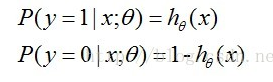我们可以将这两个式子合并得：(其中的 y = 0 或 1 )：类别为0或1 的概率函数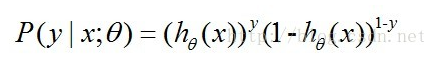这时候我们可以利用最大似然函数对向量 θ 求值，可以理解为选取的样本数据的概率是最大的

①那么样本数为 m 的似然函数为：（概率分布函数的联合分布密度）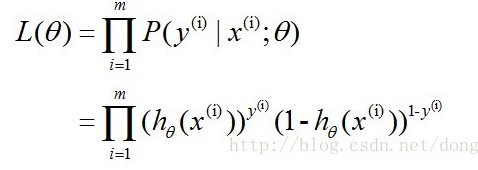②通过对数的形式对似然函数进行变化，对数似然函数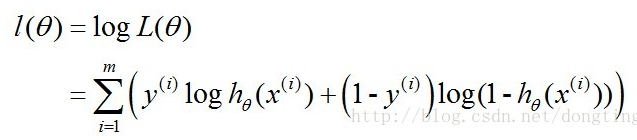此时我们不求解似然方程，而是利用梯度下降法。
这里的最大似然函数的值就是我们所要求的向量 θ , 而求解的方法利用梯度下降法。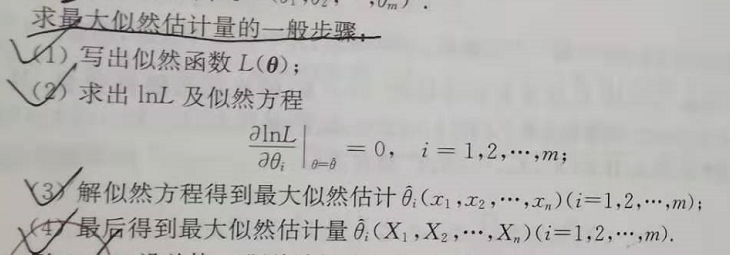### 1.4 梯度下降法求解θ

在用梯度下降法时，我们将会利用Sigmoid 函数的一个性质： g, (z) = g(z）[ 1- g(z) ] 。

构造一个Cost函数（损失函数），该函数表示预测的输出（h）与训练数据类别（y）之间的偏差。综合考虑所有训练数据的“损失”，将Cost求和或者求平均，记为J(θ)函数（代价函数），表示所有训练数据预测值与实际类别的偏差。

损失函数：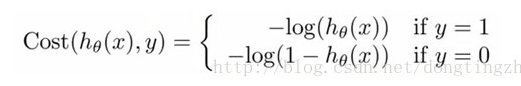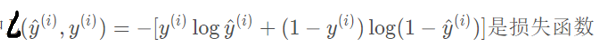J(θ)代价函数：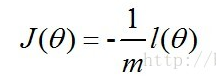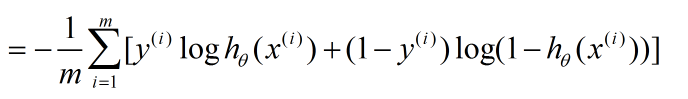其中，x(i) 每个样本数据点在某一个特征上的值，即特征向量x的某个值，y(i) 是类别号，m 是样本对象个数。

梯度下降法含义：

梯度下降法，就是利用负梯度方向来决定每次迭代的新的搜索方向，使得每次迭代能使待优化的目标函数逐步减小。梯度其实就是函数的偏导数

这里对用梯度下降法对 J (θ) 求最小值，与求似然函数的最大值是一样的。则 J(θ) 最小值的求解过程：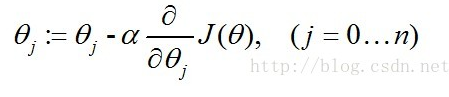其中 α 是步长。
具体步骤为：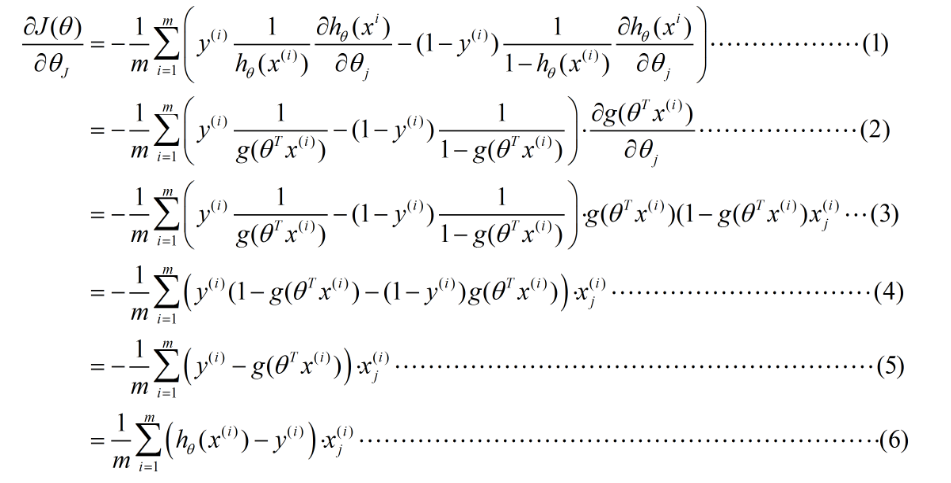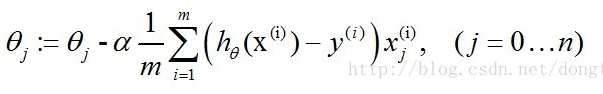随机梯度下降法，其实和批量梯度下降法原理类似，区别在与求梯度时没有用所有的m个样本的数据，而是仅仅选取一个样本j来求梯度。随机梯度下降法，和批量梯度下降法是两个极端，一个采用所有数据来梯度下降，一个用一个样本来梯度下降。自然各自的优缺点都非常突出。
对于训练速度来说，随机梯度下降法由于每次仅仅采用一个样本来迭代，训练速度很快，而批量梯度下降法在样本量很大的时候，训练速度不能让人满意。
对于准确度来说，随机梯度下降法用于仅仅用一个样本决定梯度方向，导致解很有可能不是最优。
对于收敛速度来说，由于随机梯度下降法一次迭代一个样本，导致迭代方向变化很大，不能很快的收敛到局部最优解。公式为：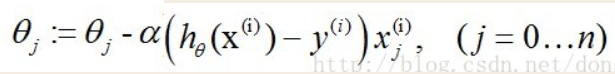到这里已经把Logistics 回归的原理推导完成。这里对以上推导做一次总结：

二分类边界线 要利用—> Sigmoid 函数 ——>要求向量 θ在 xi 确定的情况下求类别 y = 0、1 的概率—> P(y | x ; θ)  —— > 似然函数  得到—> 损失函数——>代价函数 J (θ) ——> 梯度下降法求解向量 θ ——> 最终公式 θj

步骤简略公式为：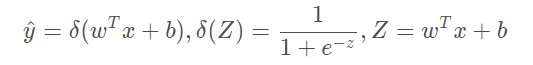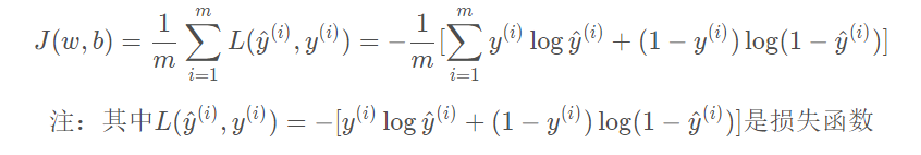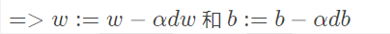2 使用python 实现Logistic 回归

from numpy import *
import matplotlib.pyplot as plt

######################################################读取数据############################################################
'''数据集的前两个值分别为X1和X2,第三个值是数据对应的类别标签，为了方便计算，把X0的值设置成了1.0'''
dataMat = []
labelMat = []
fr = open('testSet.txt')
for line in fr.readlines():
lineArr = line.strip().split()  #按行读取并按空格拆分，每一行为一个列表， ['-0.017612', '14.053064', '0']
# 为了方便计算，我们将 X0 的值设为 1.0 ，也就是在每一行的开头添加一个 1.0 作为 X0
# 因为线性回归化式为 H(x) = W0 + W1*X1 + W2*X2，即为 (W0, W1, W2)*(1.0, X1, X2),其中 (W0, W1, W2) 即为所求回归系数 W，所以模拟了一个数据列全为1.0
dataMat.append([1.0, float(lineArr), float(lineArr)])
#[[1.0, -0.017612, 14.053064], [1.0, -1.395634, 4.662541],........]含有100个元素的列表，每一个元素都为一个列表，包含3个元素
labelMat.append(int(lineArr)) #100个元素（类别标签）的列表
return dataMat, labelMat

################################################分类器的分类（转换）函数#####################################################
def sigmoid(inX):
return 1.0 / (1 + exp(-inX))

################################### 梯度上升算法，用来计算出最佳回归系数w(weights)#############################################
#输入数据特征与数据的类别标签，dataMatIn存放的是100*3的矩阵
"""
第一个参数(dataMatIn)是3维数组，每列代表每个不同特征，每行代表每个训练样本
第二个参数(labelMat)是类别标签，1*100的行向量，为便于计算，将其转换为列向量，即进行转置，并赋值给labelMat
转化矩阵[[0,1,0,1,0,1.....]]为[,,.....]
"""
dataMatrix = mat(dataMatIn) #转为100*3的矩阵 [ 1.0000000e+00 -1.7612000e-02  1.4053064e+01]
labelMat = mat(classLabels).transpose()  #转为100*1的矩阵，transpose() 行列转置函数,将行向量转化为列向量   =>  矩阵的转置
# m->数据量、样本数100    n->特征数3
m, n = shape(dataMatrix)# shape()函数是numpy.core.fromnumeric中的函数，它的功能是查看矩阵或者数组的维数
alpha = 0.001  # 步长，向函数增长最快的方向的移动量，即学习率
maxCycles = 500# 迭代次数
weights = ones((n, 1))  # 转为3*1的矩阵，元素为1的矩阵赋给weihts，即回归系数初始化为1

# 循环 maxCycles次, 每次都沿梯度向真实值 labelMat 靠拢
for k in range(maxCycles):
# 求当前sigmoid函数的值
h = sigmoid(dataMatrix * weights)  # 矩阵相乘 包含了300次的乘积 [100*3] * [3*1] = [100*1]
error = (labelMat - h)  # 向量减法，计算真实类别与预测类别的差值，h是一个列向量，列向量的元素个数等于样本数，即为100
weights = weights + alpha * dataMatrix.transpose() * error  # 矩阵相乘，dataMatrix.transpose()* error 就是梯度f(w)，按照该差值的方向调整回归系数
return weights

###################################################分析数据：画出决策边界####################################################
# 画出数据集和Logistic回归最佳拟合直线
def plotBestFit(weights):
dataMat, labelMat = loadDataSet()
dataArr = array(dataMat)
n = shape(dataArr)   # n->数据量、样本数
# xcord1,ycord1代表正例特征1,正例特征2
# xcord2,ycord2代表负例特征1,负例特征2
xcord1 = []; ycord1 = []
xcord2 = []; ycord2 = []
# 循环筛选出正负集
for i in range(n):
if int(labelMat[i]) == 1:
xcord1.append(dataArr[i, 1]); ycord1.append(dataArr[i, 2])
else:
xcord2.append(dataArr[i, 1]); ycord2.append(dataArr[i, 2])

fig = plt.figure()

# “111”表示“1×1网格，第一子图”，“234”表示“2×3网格，第四子图”。
ax.scatter(xcord1, ycord1, s=30, c='red', marker='s')
ax.scatter(xcord2, ycord2, s=30, c='green')

# 画线
# 设定边界直线x和y的值,并且以0.1为步长从-3.0到3.0切分。
x = arange(-3.0, 3.0, 0.1)#[-3.000000000 -2.90000000 -2.80000000 -2.70000000
"""
y的由来？
首先理论上是这个样子的:
dataMat.append([1.0, float(lineArr), float(lineArr)])
w0*x0+w1*x1+w2*x2=f(x)
x0最开始就设置为1， x2就是我们画图的y值。0是两个类别的分界处
所以： w0+w1*x+w2*y=0 => y = (-w0-w1*x)/w2
"""
y = (-weights - weights * x) / weights  #最佳拟合直线

ax.plot(x, y)
plt.xlabel('X1');
plt.ylabel('X2');
plt.show()

# 输出运用梯度上升优化算法后得到的最理想的回归系数的值
if __name__ =='__main__':
plotBestFit(weights.getA())


数据集为

-0.017612	14.053064	0
-1.395634	4.662541	1
-0.752157	6.538620	0
-1.322371	7.152853	0
0.423363	11.054677	0
0.406704	7.067335	1
0.667394	12.741452	0
-2.460150	6.866805	1
0.569411	9.548755	0
-0.026632	10.427743	0
0.850433	6.920334	1
1.347183	13.175500	0
1.176813	3.167020	1
-1.781871	9.097953	0
-0.566606	5.749003	1
0.931635	1.589505	1
-0.024205	6.151823	1
-0.036453	2.690988	1
-0.196949	0.444165	1
1.014459	5.754399	1
1.985298	3.230619	1
-1.693453	-0.557540	1
-0.576525	11.778922	0
-0.346811	-1.678730	1
-2.124484	2.672471	1
1.217916	9.597015	0
-0.733928	9.098687	0
-3.642001	-1.618087	1
0.315985	3.523953	1
1.416614	9.619232	0
-0.386323	3.989286	1
0.556921	8.294984	1
1.224863	11.587360	0
-1.347803	-2.406051	1
1.196604	4.951851	1
0.275221	9.543647	0
0.470575	9.332488	0
-1.889567	9.542662	0
-1.527893	12.150579	0
-1.185247	11.309318	0
-0.445678	3.297303	1
1.042222	6.105155	1
-0.618787	10.320986	0
1.152083	0.548467	1
0.828534	2.676045	1
-1.237728	10.549033	0
-0.683565	-2.166125	1
0.229456	5.921938	1
-0.959885	11.555336	0
0.492911	10.993324	0
0.184992	8.721488	0
-0.355715	10.325976	0
-0.397822	8.058397	0
0.824839	13.730343	0
1.507278	5.027866	1
0.099671	6.835839	1
-0.344008	10.717485	0
1.785928	7.718645	1
-0.918801	11.560217	0
-0.364009	4.747300	1
-0.841722	4.119083	1
0.490426	1.960539	1
-0.007194	9.075792	0
0.356107	12.447863	0
0.342578	12.281162	0
-0.810823	-1.466018	1
2.530777	6.476801	1
1.296683	11.607559	0
0.475487	12.040035	0
-0.783277	11.009725	0
0.074798	11.023650	0
-1.337472	0.468339	1
-0.102781	13.763651	0
-0.147324	2.874846	1
0.518389	9.887035	0
1.015399	7.571882	0
-1.658086	-0.027255	1
1.319944	2.171228	1
2.056216	5.019981	1
-0.851633	4.375691	1
-1.510047	6.061992	0
-1.076637	-3.181888	1
1.821096	10.283990	0
3.010150	8.401766	1
-1.099458	1.688274	1
-0.834872	-1.733869	1
-0.846637	3.849075	1
1.400102	12.628781	0
1.752842	5.468166	1
0.078557	0.059736	1
0.089392	-0.715300	1
1.825662	12.693808	0
0.197445	9.744638	0
0.126117	0.922311	1
-0.679797	1.220530	1
0.677983	2.556666	1
0.761349	10.693862	0
-2.168791	0.143632	1
1.388610	9.341997	0
0.317029	14.739025	0

### 2.3 利用随机梯度上升法训练样本

from numpy import *
import matplotlib.pyplot as plt
import numpy as np

######################################################读取数据############################################################
'''数据集的前两个值分别为X1和X2,第三个值是数据对应的类别标签，为了方便计算，把X0的值设置成了1.0'''
dataMat = []
labelMat = []
fr = open('testSet.txt')
for line in fr.readlines():
lineArr = line.strip().split()  #按行读取并按空格拆分，每一行为一个列表， ['-0.017612', '14.053064', '0']
# 为了方便计算，我们将 X0 的值设为 1.0 ，也就是在每一行的开头添加一个 1.0 作为 X0
# 因为线性回归化式为 H(x) = W0 + W1*X1 + W2*X2，即为 (W0, W1, W2)*(1.0, X1, X2),其中 (W0, W1, W2) 即为所求回归系数 W，所以模拟了一个数据列全为1.0
dataMat.append([1.0, float(lineArr), float(lineArr)])
#[[1.0, -0.017612, 14.053064], [1.0, -1.395634, 4.662541],........]含有100个元素的列表，每一个元素都为一个列表，包含3个元素
labelMat.append(int(lineArr)) #100个元素（类别标签）的列表
return dataMat, labelMat

################################################分类器的分类（转换）函数#####################################################
def sigmoid(inX):
return 1.0 / (1 + exp(-inX))

def stocGradAscent1(dataMatrix, classLabels, numIter=150):
dataMatrix = np.array(dataMatrix)
m, n = np.shape(dataMatrix)  # 返回dataMatrix的大小。m为行数,n为列数。
weights = np.ones(n)  # 参数初始化
for j in range(numIter):
dataIndex = list(range(m))
for i in range(m):
alpha = 4 / (1.0 + j + i) + 0.01  # 降低alpha的大小，每次减小1/(j+i)。
randIndex = int(random.uniform(0, len(dataIndex)))  # 随机选取样本
h = sigmoid(sum(dataMatrix[randIndex] * weights))  # 选择随机选取的一个样本，计算h
error = classLabels[randIndex] - h  # 计算误差
weights = weights + alpha * error * dataMatrix[randIndex]  # 更新回归系数
del (dataIndex[randIndex])  # 删除已经使用的样本
return weights  # 返回

###################################################分析数据：画出决策边界####################################################
# 画出数据集和Logistic回归最佳拟合直线
def plotBestFit(weights):
dataMat, labelMat = loadDataSet()
dataArr = array(dataMat)
n = shape(dataArr)   # n->数据量、样本数
# xcord1,ycord1代表正例特征1,正例特征2
# xcord2,ycord2代表负例特征1,负例特征2
xcord1 = []; ycord1 = []
xcord2 = []; ycord2 = []
# 循环筛选出正负集
for i in range(n):
if int(labelMat[i]) == 1:
xcord1.append(dataArr[i, 1]); ycord1.append(dataArr[i, 2])
else:
xcord2.append(dataArr[i, 1]); ycord2.append(dataArr[i, 2])

fig = plt.figure()

# “111”表示“1×1网格，第一子图”，“234”表示“2×3网格，第四子图”。
ax.scatter(xcord1, ycord1, s=30, c='red', marker='s')
ax.scatter(xcord2, ycord2, s=30, c='green')

# 画线
# 设定边界直线x和y的值,并且以0.1为步长从-3.0到3.0切分。
x = arange(-3.0, 3.0, 0.1)#[-3.000000000 -2.90000000 -2.80000000 -2.70000000
"""
y的由来？
首先理论上是这个样子的:
dataMat.append([1.0, float(lineArr), float(lineArr)])
w0*x0+w1*x1+w2*x2=f(x)
x0最开始就设置为1， x2就是我们画图的y值。0是两个类别的分界处
所以： w0+w1*x+w2*y=0 => y = (-w0-w1*x)/w2
"""
y = (-weights - weights * x) / weights  #最佳拟合直线

ax.plot(x, y)
plt.xlabel('X1');
plt.ylabel('X2');
plt.show()

# 输出运用梯度上升优化算法后得到的最理想的回归系数的值
if __name__ =='__main__':
plotBestFit(weights)

展开全文机器学习
•logistics
• Logistics回归：极大似然法 对数几率 因此有： Logisitics回归：极大似然法估计recap 确定待求解的未知参数θ1，θ2，……θn，如均值，方差或特定分布函数等 计算每个样本X1 , X2 , . . . , Xn的概率密度 f(Xi; θ1...

Logistics回归：极大似然法

对数几率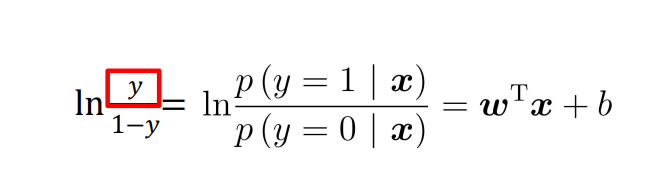因此有：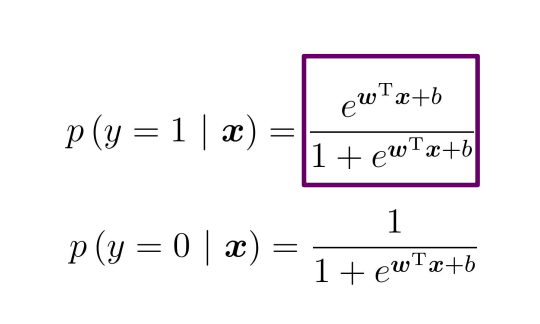Logisitics回归：极大似然法估计recap

确定待求解的未知参数θ1，θ2，……θn，如均值，方差或特定分布函数等

计算每个样本X1 , X2 , . . . , Xn的概率密度 f(Xi; θ1 , . . . , θm).

假定样本i.i.d，则可根据样本的概率密度累乘构造似然函数：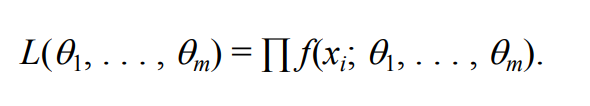通过似然函数最大化（求导为零），求解未知参数 θ

为降低计算难度，通常可采用对数加法替换概率乘法，通 过导数为零/极大值来求解未知参数

UCI Machine Learning Repository: Breast Cancer Wisconsin (Original) Data Set

数据库借用的康威斯星州乳腺癌的数据集

我将其中的10与8改成了0，增加数据集数据错误率

1.改进的随机梯度上升算法

def stocGradAscent1(dataMatrix, classLabels, numIter=150):
m,n = shape(dataMatrix)
weights = ones(n)   #initialize to all ones
for j in range(numIter):
dataIndex = range(m)
for i in range(m):
alpha = 4/(1.0+j+i)+0.0001    #apha decreases with iteration, does not
randIndex = int(random.uniform(0,len(dataIndex)))#go to 0 because of the constant
h = sigmoid(sum(dataMatrix[randIndex]*weights))
error = classLabels[randIndex] - h
weights = weights + alpha * error * dataMatrix[randIndex]
del(dataIndex[randIndex])
return weights

def classifyVector(inX, weights):
prob = sigmoid(sum(inX*weights))
if prob > 0.5: return 1.0
else: return 0.0

def colicTest():
frTrain = open('breast-cancer-wisconsin.txt'); frTest = open('breast-cancer-wisconsin-train.txt')
trainingSet = []; trainingLabels = []
for line in frTrain.readlines():
currLine = line.strip().split(',')
lineArr =[]
for i in range(10):
lineArr.append(float(currLine[i]))
trainingSet.append(lineArr)
trainingLabels.append(float(currLine))
trainWeights = stocGradAscent1(array(trainingSet), trainingLabels, 1000)
errorCount = 0; numTestVec = 0.0
for line in frTest.readlines():
numTestVec += 1.0
currLine = line.strip().split(',')
lineArr =[]
for i in range(10):
lineArr.append(float(currLine[i]))
if int(classifyVector(array(lineArr), trainWeights))!= int(currLine):
errorCount += 1
errorRate = (float(errorCount)/numTestVec)
print "the error rate of this test is: %f" % errorRate
return errorRate

def multiTest():
numTests = 10; errorSum=0.0
for k in range(numTests):
errorSum += colicTest()
print "after %d iterations the average error rate is: %f" % (numTests, errorSum/float(numTests))

输出结果为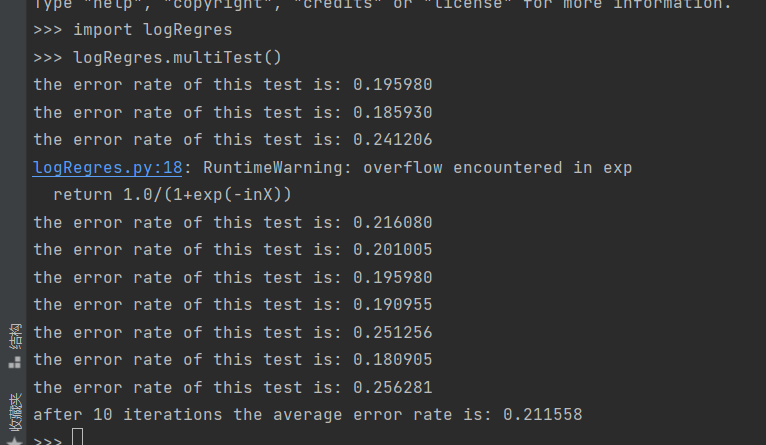0（即未收集数据）占总数据的百分比约为13%，而利用改进的随机梯度算法错误率仅仅20%，可以说效率非常高

2.随机梯度上升算法

def stocGradAscent0(dataMatrix, classLabels):
m,n = shape(dataMatrix)
alpha = 0.01
weights = ones(n)   #initialize to all ones
for i in range(m):
h = sigmoid(sum(dataMatrix[i]*weights))
error = classLabels[i] - h
weights = weights + alpha * error * dataMatrix[i]
return weights


利用此算法计算的错误率结果为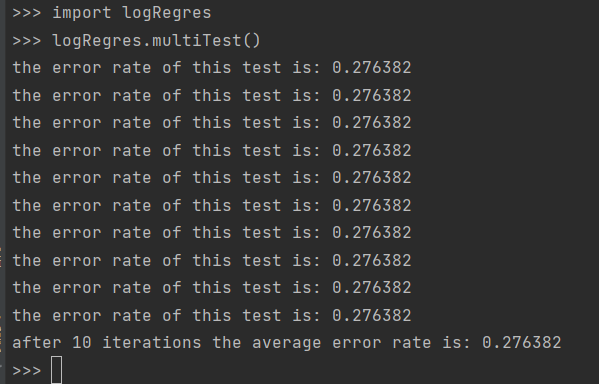可以看出相比改进的随机梯度上升算法来看，错误率明显提高不少

所以改进的随机梯度上升算法更好

展开全文机器学习
• 因为自己对回归分类比较好奇，所以就找了找资料做了这篇笔记。 一、什么是回归？ 这个问题对我来说一直很朦胧，因为虽然之前做过一些回归的实验，但是如果真是让我用语言说出来又不知道说什么，所以就查了查资料。 ...分类 机器学习
• Logistic回归虽然名字叫”回归” ，但却是一种分类学习方法。使用场景大概有两个：第一用来预测，第二寻找因变量的影响因素。 一、从线性回归到Logistic回归 线性回归和Logistic回归都是广义线性模型的特例。 假设有...hadoop 数据挖掘 机器学习
• 数据回归-老年关节置换患者发生术后谵妄的多因素logistics回归分析.pdf
• 该文章只是方便我学习机器学习，若有错误之处，请帮忙斧正。
• Logistics回归、Softmax代码实现–Tensorflow部分–潘登同学的机器学习笔记 python版本–3.6 ; Tensorflow版本–1.15.0 ;编辑器–Pycharm 文章目录Logistics回归、Softmax代码实现--Tensorflow部分--潘登同学的机器...tensorflow python
• 线性回归对于分类问题 线性回归解决的是回归问题，即连续变量的问题，在直角坐标系下就是用直线去拟合数据点；虽然在分类问题中也可以用线性...logistics回归原理 对于二分类问题，我们要预测“正”、“反”两种结...
• 基于贝叶斯理论的logistic回归模型的建立、预测。
• Python使用Logistics回归进行分类 代码 #Logistics回归分类 import pandas as pd from sklearn.linear_model import LogisticRegression # 导入sklearn库的LogisticRegression函数 from sklearn.model_selection ...机器学习 python sklearn
• opencv3.4.1+vs2019 机器学习练习，手写字符训练。 函数接口：输入路径进行训练，输入图像进行预测，预测输出分类的分数 程序包括数据集和模型，模型文件为data\..xml机器学习
• Logistics回归定义 说是回归，但其本质上还是属于分类算法。利用Logistics回归进行分类的主要思想是：根据现有数据对分类边界建立回归方程，以此进行分类。 基于Logistics回归和Sigmoid函数的分类 因为要分类，于是...
• 1. Logistics回归与线性回归 线性回归就是给定n个变量x，经过一些线性组合后得到一个预测值y，而Logistics回归实际则是一个二分类问题，将线性回归预测的值通过一个sigmod函数，编程了（0,1）之间的概率，然后规定...
• 多元线性回归及logistics回归模型在上海市房价预测中的分析与应用.rar
• 广义线性回归 ?glm data(breslow.dat, package="robust") names(breslow.dat) summary(breslow.dat[c(6，7，8，10)]) attach(breslow.dat) > fit <-glm(sumY ~ Base + Age + Trt,data=breslow.dat, ...r语言
• 今天主要整理，吴恩达机器学习的logistics 回归这一节的笔记吴恩达 机器学习
• 多元线性回归及logistics回归模型在上海市房价预测中的分析与应用.doc
• 多元logistics回归分析PPT学习教案.pptx...